# Chapter 17 Amortized Analysis III 1 Dynamic Table

• Slides: 22Chapter 17: Amortized Analysis III 1Dynamic Table • Sometimes, we may not know in advance the #objects to be stored in a table • We may allocate space for the table, say with malloc(), at the beginning • When more objects are inserted, the space allocated before may not be enough 2Dynamic Table • The table must then be reallocated with a larger size, say with realloc(), and all objects from original table must be copied over into the new, larger table • Similarly, if many objects are deleted, we may want to reallocate the table with a smaller size (to save space) • Any good choice for the reallocation size? 3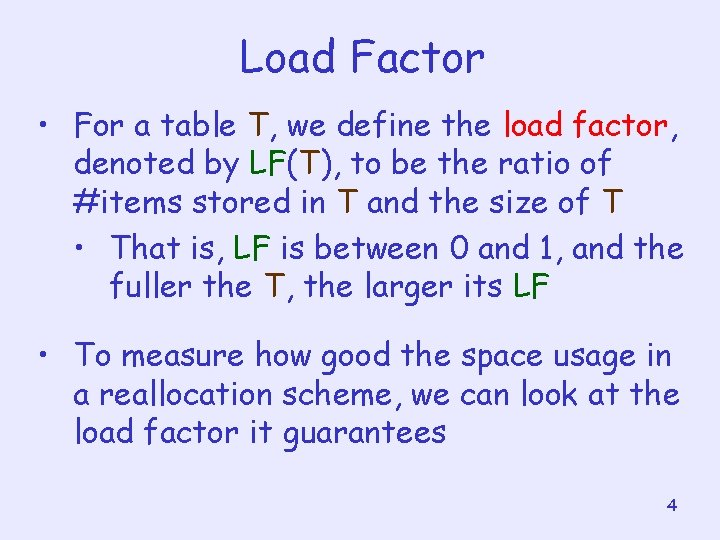Load Factor • For a table T, we define the load factor, denoted by LF(T), to be the ratio of #items stored in T and the size of T • That is, LF is between 0 and 1, and the fuller the T, the larger its LF • To measure how good the space usage in a reallocation scheme, we can look at the load factor it guarantees 4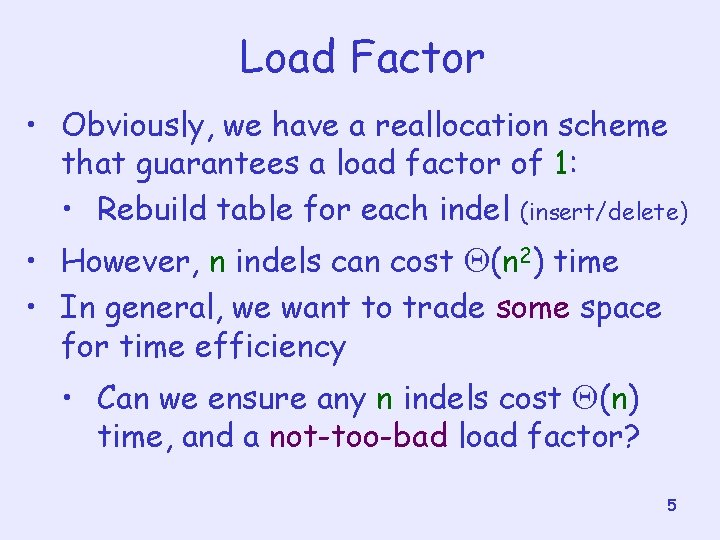Load Factor • Obviously, we have a reallocation scheme that guarantees a load factor of 1: • Rebuild table for each indel (insert/delete) • However, n indels can cost Q(n 2) time • In general, we want to trade some space for time efficiency • Can we ensure any n indels cost Q(n) time, and a not-too-bad load factor? 5Handling Insertion • Suppose we have only insertion operations • Our reallocation scheme is as follows: If T is full before insertion of an item, we expand T by doubling its size • It is clear that at any time, LF(T) is at least 0. 5 • Question: How about the insertion cost? 6Handling Insertion • Observe that the more items are stored, the closer the next expansion will come • Let num(T) = #items currently stored in T • Let size(T) = size of T • To reflect this observation, we define a potential function such that (T) = 2 num(T) – size(T) 7Handling Insertion • The function has some nice properties: • Immediately before an expansion, (T) = num(T) = size (T) this provides enough cost to copy items into new table • Immediately after an expansion, (T) = 0 this resets everything, and simplify the analysis • Its value is always non-negative is always at least half full) (the table 8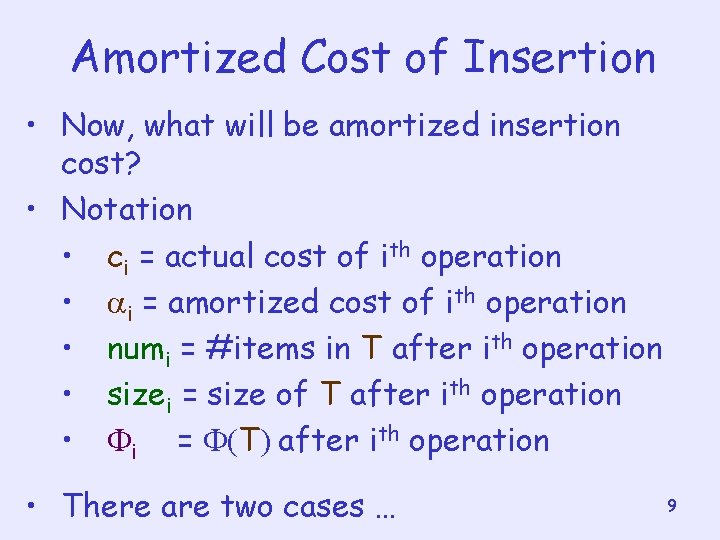Amortized Cost of Insertion • Now, what will be amortized insertion cost? • Notation • ci = actual cost of ith operation • i = amortized cost of ith operation • numi = #items in T after ith operation • sizei = size of T after ith operation • i = (T) after ith operation • There are two cases … 9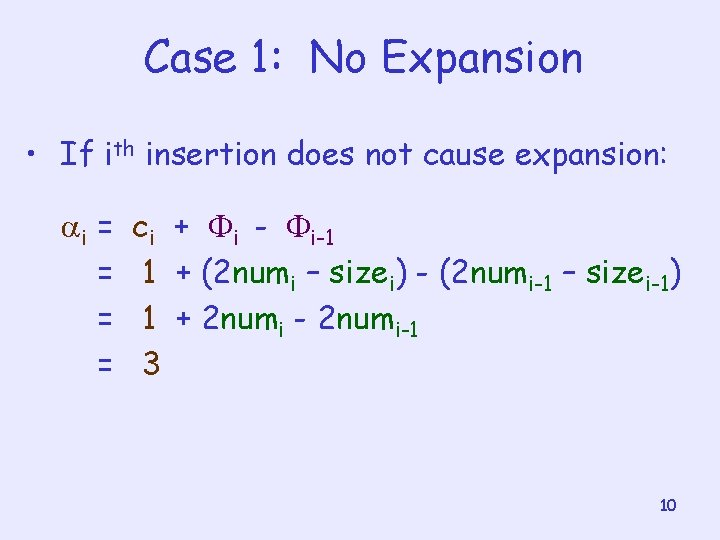Case 1: No Expansion • If ith insertion does not cause expansion: i = = ci + i - i-1 1 + (2 numi – sizei) - (2 numi-1 – sizei-1) 1 + 2 numi - 2 numi-1 3 10Case 2: With Expansion • If ith insertion causes an expansion: sizei =2 sizei-1 and sizei-1 =numi-1 = numi – 1 i = ci + i - i-1 = numi + (2 numi – sizei) - (2 numi-1 – sizei-1) = numi + (2 numi– 2(numi – 1)) - (2(numi – 1) – (numi – 1)) = numi + 2 - (numi – 1) =3 Conclusion: amortized cost for insertion = O(1) 11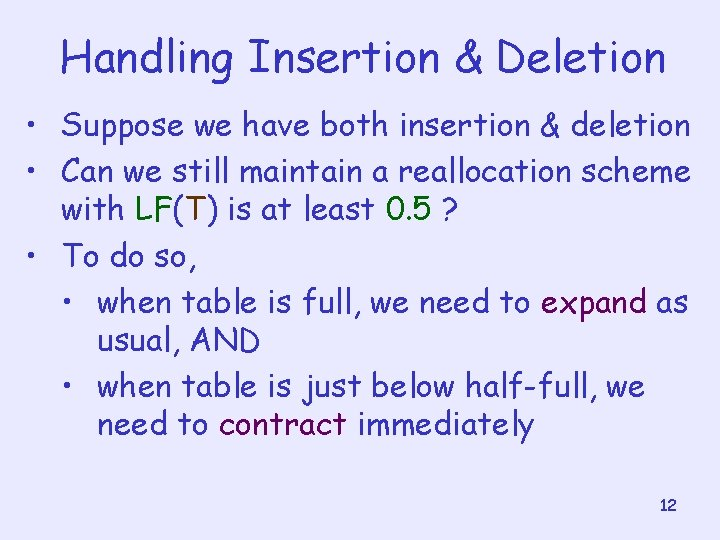Handling Insertion & Deletion • Suppose we have both insertion & deletion • Can we still maintain a reallocation scheme with LF(T) is at least 0. 5 ? • To do so, • when table is full, we need to expand as usual, AND • when table is just below half-full, we need to contract immediately 12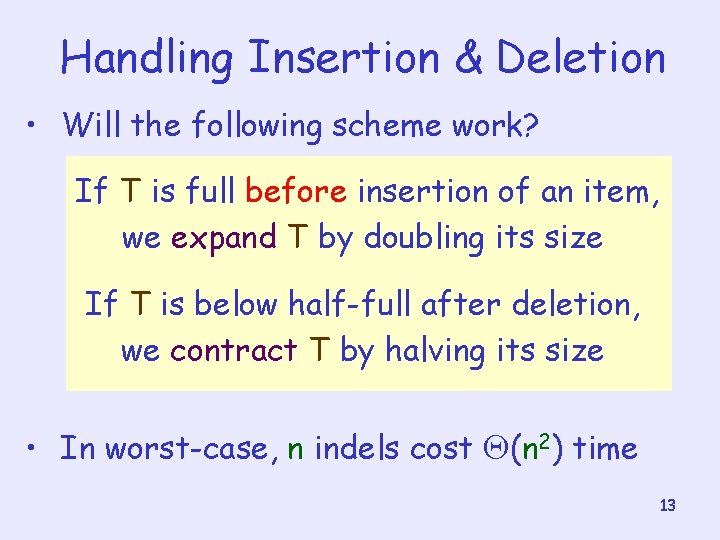Handling Insertion & Deletion • Will the following scheme work? If T is full before insertion of an item, we expand T by doubling its size If T is below half-full after deletion, we contract T by halving its size • In worst-case, n indels cost Q(n 2) time 13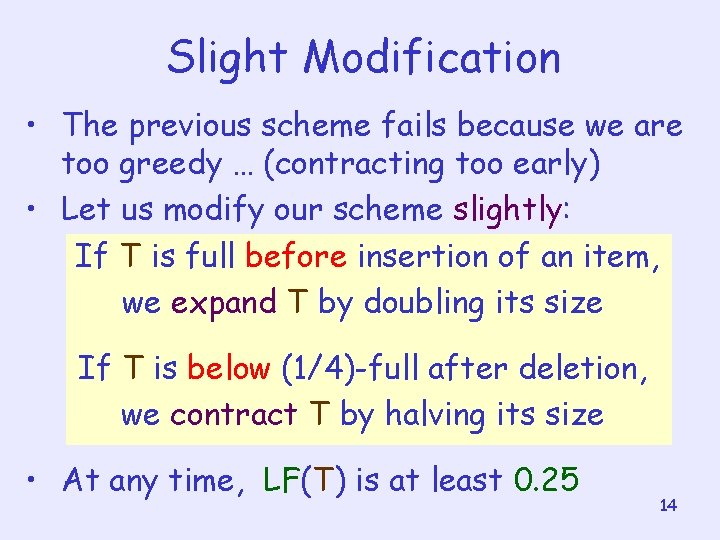Slight Modification • The previous scheme fails because we are too greedy … (contracting too early) • Let us modify our scheme slightly: If T is full before insertion of an item, we expand T by doubling its size If T is below (1/4)-full after deletion, we contract T by halving its size • At any time, LF(T) is at least 0. 25 14Handling Insertion & Deletion • Now, using this scheme, • If table is more than (1/2)-full, we should start worrying about the next expansion watch for insertion • If table is less than (1/2)-full, we should start worrying about the next contraction watch for deletion • This gives us some intuition of how to define the potential function 15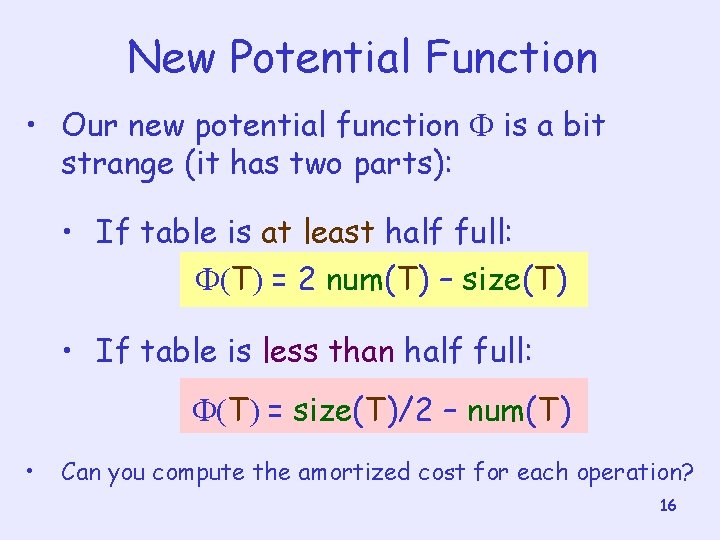New Potential Function • Our new potential function is a bit strange (it has two parts): • If table is at least half full: (T) = 2 num(T) – size(T) • If table is less than half full: (T) = size(T)/2 – num(T) • Can you compute the amortized cost for each operation? 16Nice Properties • The function has some nice properties: • Immediately before a resize, (T) = num(T) this provides enough cost to copy items into new table • At half-full, (T) = 0 this resets everything, and simplify the analysis • Its value is always non-negative 17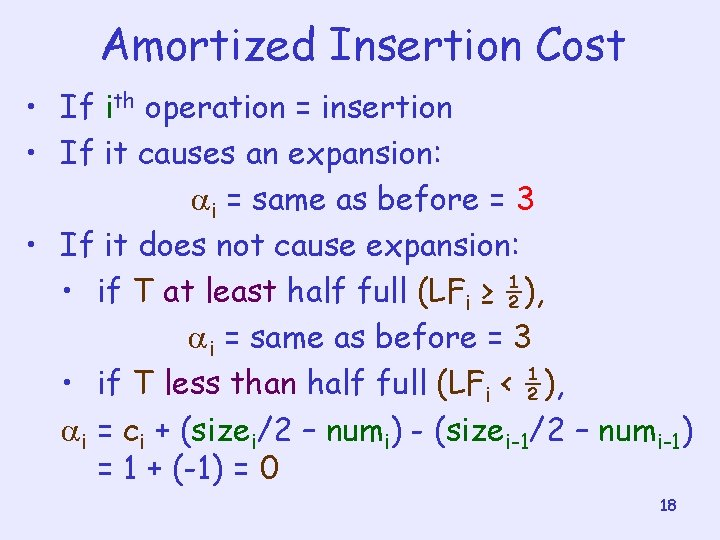Amortized Insertion Cost • If ith operation = insertion • If it causes an expansion: i = same as before = 3 • If it does not cause expansion: • if T at least half full (LFi ≥ ½), i = same as before = 3 • if T less than half full (LFi < ½), i = ci + (sizei/2 – numi) - (sizei-1/2 – numi-1) = 1 + (-1) = 0 18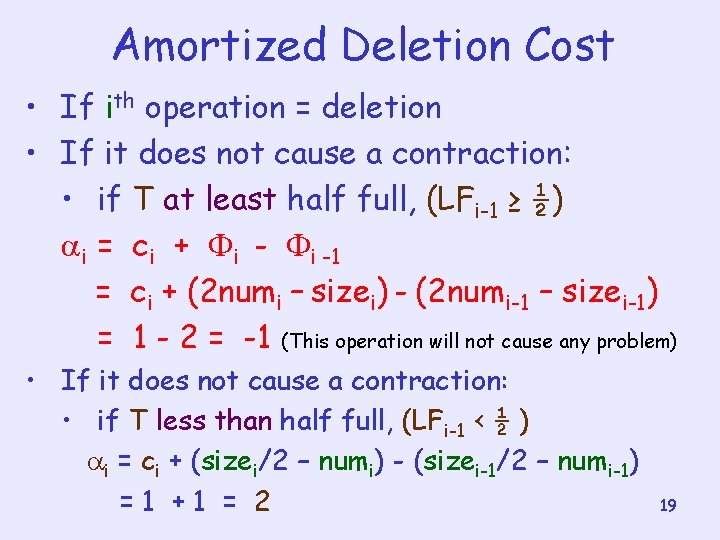Amortized Deletion Cost • If ith operation = deletion • If it does not cause a contraction: • if T at least half full, (LFi-1 ≥ ½) i = ci + i -1 = ci + (2 numi – sizei) - (2 numi-1 – sizei-1) = 1 - 2 = -1 (This operation will not cause any problem) • If it does not cause a contraction: • if T less than half full, (LFi-1 < ½ ) i = ci + (sizei/2 – numi) - (sizei-1/2 – numi-1) =1 +1 = 2 19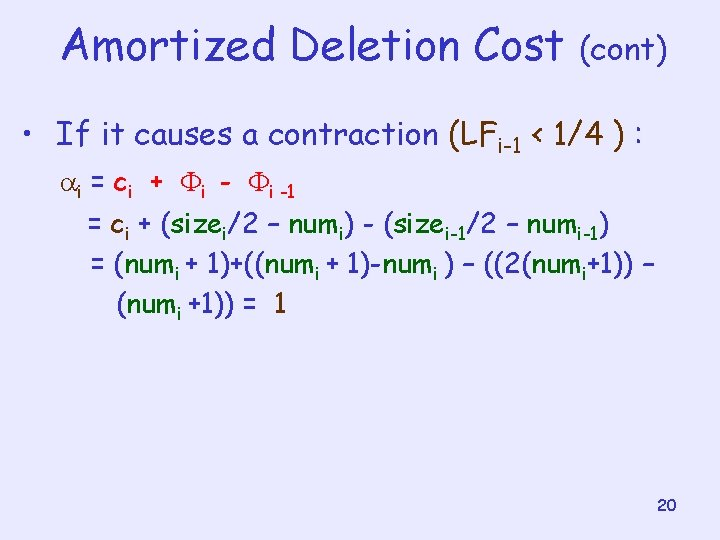Amortized Deletion Cost (cont) • If it causes a contraction (LFi-1 < 1/4 ) : i = ci + i -1 = ci + (sizei/2 – numi) - (sizei-1/2 – numi-1) = (numi + 1)+((numi + 1)-numi ) – ((2(numi+1)) – (numi +1)) = 1 20Conclusion • The amortized insertion or deletion cost in our new scheme = O(1) • Meaning: Any n operations in total cost O(n) time • Remark: There can be other reallocation schemes with O(1) load factor and O(1) amortized cost (Try to think about it !) 21Homework • • Exercise: 17. 4 -2, 17. 4 -3 (Due: Dec. 5) Practice ay home: 17. 4 -1 Second Mid-Term Exam. Dec. 14 Ch 19 Fibonacci heaps (Self-study) 22